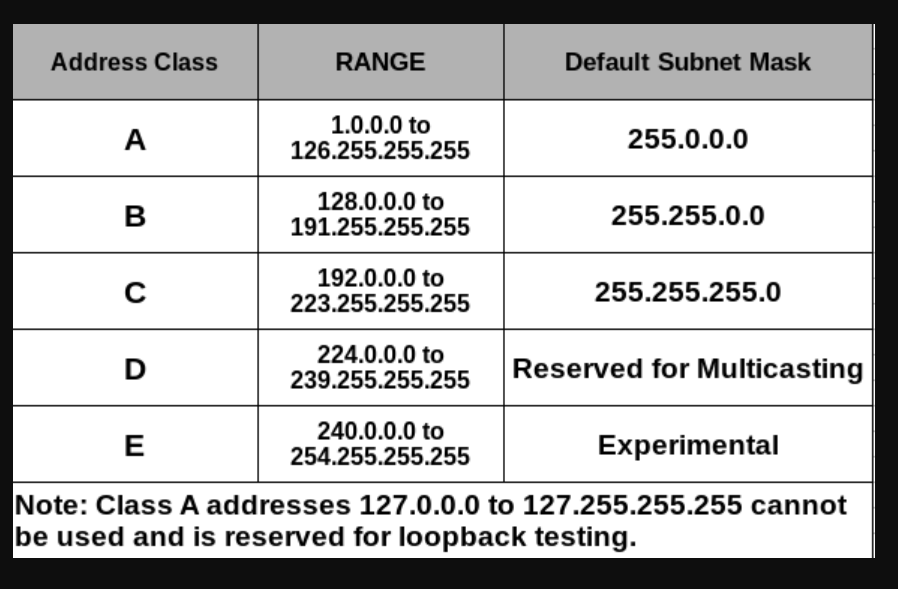## Networking Basics

### Problem Statement – Network Design

#### Problem 1

• we need a network to connect 200 devices• We need to figure out the network id and host id ranges
• Generally we have two kinds of networks
• public network:
• These ip addresses are accessible over internet
• We have very limited public ip’s available in IPv4
• private network
• These ip addresses are reserved for intranet
• The private ip addresses which are reserved are
• 10.0.0.0 to 10.255.255.255
• 172.16.0.0 to 172.31.255.255
• 192.168.0.0 to 192.168.255.255
• Note: When we create network through out the exercises as well as in Azure we will be using private ranges.
• Solution
``````Subnet Mask: 255.255.255.0 => 1 octect => 8 => 2^8 = 256
255.255.0.0 => 2 octects => 16 => 2^16 = 65536
255.0.0.0 => 3 octects => 24 => 2^24 => 16777216

private ranges:
10.0.0.0 to 10.255.255.255
172.16.0.0 to 172.31.255.255
192.168.0.0 to 192.168.255.255

Size ~= 200
SM = 255.255.255.0
some possibilties
ip: 10.0.0.x
sm: 255.255.255.0

ip: 10.0.1.x
sm: 255.255.255.0

ip: 192.168.0.x
sm: 255.255.255.0
``````

#### Problem 2

• We need a network to connect 500000 devices
``````Subnet Mask: 255.255.255.0 => 1 octect => 8 => 2^8 = 256
255.255.0.0 => 2 octects => 16 => 2^16 = 65536
255.0.0.0 => 3 octects => 24 => 2^24 => 16777216

private ranges:
10.0.0.0 to 10.255.255.255
172.16.0.0 to 172.31.255.255
192.168.0.0 to 192.168.255.255

Size = 5,00,000

IP: 10.x.x.x
SM: 255.0.0.0
``````

### Problem 3

• I need a network to connect 500 devices
``````Subnet Mask: 255.255.255.0 => 1 octect => 8 => 2^8 = 256
255.255.0.0 => 2 octects => 16 => 2^16 = 65536
255.0.0.0 => 3 octects => 24 => 2^24 => 16777216

private ranges:
10.0.0.0 to 10.255.255.255
172.16.0.0 to 172.31.255.255
192.168.0.0 to 192.168.255.255

Size = 500

IP: 10.0.x.x
SM = 255.255.0.0

IP: 172.16.x.x
SM = 255.255.0.0

IP: 192.168.x.x
SM = 255.255.0.0

``````
• Till now the way we are creating networks i.e. we want a network for 500 but we had to choose 65000 size network

• We have two options
• Classful:
• We have classes of networks• Classless:
• We try looking at subnet mask as binary number

This site uses Akismet to reduce spam. Learn how your comment data is processed.